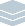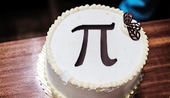Wolfram Archive
Wolfram Programming Lab is a legacy product.
All the same functionality and features, including access to Programming Lab Explorations, are available with Wolfram|One.
Start programming now. »
Try it now »
(no sign-in required)# Find Your Birthday in Pi

Find where in the digits of Pi the digits of your birthday occur.

Run the code to get the first 1,000 digits of Pi. Try more digits:

Get a list of the first 20 digits of Pi in base 10, plus the number of digits to the left of the decimal point:

RealDigits[Pi, 10, 20]

Get just the list of digits:

First[RealDigits[Pi, 10, 20]]

First[RealDigits[Pi, 10, 1000]]

Find where your birthday occurs in the digits of Pi. Try other sequences of digits:

Note: increase the number of digits of Pi until you find your sequence; e.g. try 10 times more digits each time.

Note: sequences of digits longer than 6 may require a very long time to compute.

Make a list of the digits of your birthdayfor example, for November 17, 1999:

{1, 1, 1, 7, 9, 9}

Find the position of that sequence in the digits of Pi:

SequencePosition[ First[RealDigits[Pi, 10, 1000000]], {1, 1, 1, 7, 9, 9}]

The pairs of numbers are the positions in the digits of Pi of the first and last digits of the sequence. If the sequence occurs more than once, youll get more than one pair. If the sequence doesnt occur at all, youll get the empty list {}.

Increase the number of digits of Pi if your sequence is not found. For example, try 10 times more digits.

SequencePosition[First[RealDigits[Pi, 10, 1000000]], {1, 2, 3, 4, 5}]

Print the digits of Pi, ending at the first occurrence of your birthday:

Find the position of your birthday in the digits of Pi. Increase the number of digits, if necessary, to find the sequence:

SequencePosition[First[RealDigits[Pi, 10, 1000000]], {1, 2, 3, 4, 5}]

The end of the first occurrence of the sequence is 49,707. Get the first 49,707 digits of Pi and give it the name digits:

digits = First[RealDigits[Pi, 10, 49707]];

49,707 digits are way too many to print, so instead make an abbreviated form.

This gives the first 100 digits:

digits[[1 ;; 100]]

This gives the last 100 digits (-1 means the last digit, -100 means the 100th-from-last digit):

digits[[-100 ;; -1]]

Row formats a list of objects without the braces, commas, and quotation marksfor example:

Row[{"The ", 5, "th prime is ", 11, "."}]

Use Row to show the first 100 digits, an ellipsis (...), and the last 100 digits. Flatten flattens out the sublists of digits:

Row[Flatten[{digits[[1 ;; 100]], "...", digits[[-100 ;; -1]]}]]

Restore the decimal point by omitting the first digit and supplying the missing 3 and decimal point explicitly:

Row[Flatten[{"3.", digits[[2 ;; 100]], "...", digits[[-100 ;; -1]]}]]

This code is essentially what was implemented to make the Wolfram My Pi Day site.

digits = First[RealDigits[Pi, 10, 49707]]; Row[Flatten[{"3.", digits[[2 ;; 100]], "...", digits[[-100 ;; -1]]}]]